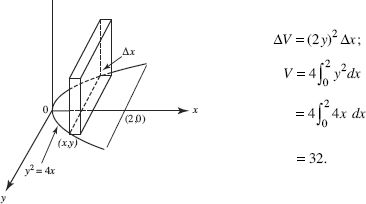# AP Calculus BC Question 162: Answer and Explanation

### Test Information

Question: 162

8. The base of a solid is the region bounded by the parabola y2 = 4x and the line x = 2. Each plane section perpendicular to the x-axis is a square. The volume of the solid is

• A. 32
• B. 8
• C. 10
• D. 16